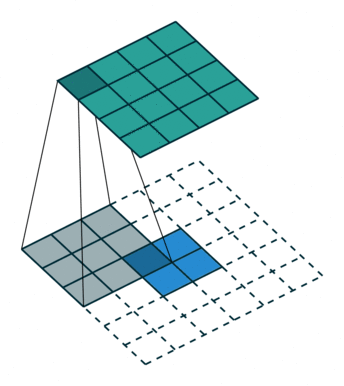# 4. Dive deep into Training a Simple Pose Model on COCO Keypoints¶

In this tutorial, we show you how to train a pose estimation model 1 on the COCO dataset.

First let’s import some necessary modules.

from __future__ import division

import time, logging, os, math

import numpy as np
import mxnet as mx
from mxnet import gluon, nd
from mxnet import autograd as ag
from mxnet.gluon import nn
from mxnet.gluon.data.vision import transforms

from gluoncv.data import mscoco
from gluoncv.model_zoo import get_model
from gluoncv.utils import makedirs, LRScheduler
from gluoncv.data.transforms.presets.simple_pose import SimplePoseDefaultTrainTransform
from gluoncv.utils.metrics import HeatmapAccuracy


We can load COCO Keypoints dataset with their official API

train_dataset = mscoco.keypoints.COCOKeyPoints('~/.mxnet/datasets/coco',
splits=('person_keypoints_train2017'))


The dataset object enables us to retrieve images containing a person, the person’s keypoints, and meta-information.

Following the original paper, we resize the input to be (256, 192). For augmentation, we randomly scale, rotate or flip the input. Finally we normalize it with the standard ImageNet statistics.

The COCO keypoints dataset contains 17 keypoints for a person. Each keypoint is annotated with three numbers (x, y, v), where x and y mark the coordinates, and v indicates if the keypoint is visible.

For each keypoint, we generate a gaussian kernel centered at the (x, y) coordinate, and use it as the training label. This means the model predicts a gaussian distribution on a feature map.

transform_train = SimplePoseDefaultTrainTransform(num_joints=train_dataset.num_joints,
joint_pairs=train_dataset.joint_pairs,
image_size=(256, 192), heatmap_size=(64, 48),
scale_factor=0.30, rotation_factor=40, random_flip=True)


Now we can define our data loader with the dataset and transformation. We will iterate over train_data in our training loop.

batch_size = 32
train_dataset.transform(transform_train),


## Deconvolution Layer¶

A deconvolution layer enlarges the feature map size of the input, so that it can be seen as a layer upsamling the input feature map.In the above image, the blue map is the input feature map, and the cyan map is the output.

In a ResNet model, the last feature map shrinks its height and width to be only 1/32 of the input. It may be too small for a heatmap prediction. However if followed by several deconvolution layers, the feature map can have a larger size thus easier to make the prediction.

## Model Definition¶

A Simple Pose model consists of a main body of a resnet, and several deconvolution layers. Its final layer is a convolution layer predicting one heatmap for each keypoint.

Let’s take a look at the smallest one from the GluonCV Model Zoo, using ResNet18 as its base model.

We load the pre-trained parameters for the ResNet18 layers, and initialize the deconvolution layer and the final convolution layer.

context = mx.gpu(0)
net = get_model('simple_pose_resnet18_v1b', num_joints=17, pretrained_base=True,
ctx=context, pretrained_ctx=context)
net.deconv_layers.initialize(ctx=context)
net.final_layer.initialize(ctx=context)


We can take a look at the summary of the model

x = mx.nd.ones((1, 3, 256, 192), ctx=context)
net.summary(x)


Note

The Batch Normalization implementation from cuDNN has a negative impact on the model training, as reported in these issues 2, 3 .

Since similar behavior is observed, we implement a BatchNormCudnnOff layer as a temporary solution. This layer doesn’t call the Batch Normalization layer from cuDNN, thus gives better results.

## Training Setup¶

Next, we can set up everything for the training.

• Loss:

We apply a weighted L2Loss on the predicted heatmap, where the weight is 1 if the keypoint is visible, otherwise is 0.

L = gluon.loss.L2Loss()

• Learning Rate Schedule and Optimizer:

We use an initial learning rate at 0.001, and divide it by 10 at the 90th and 120th epoch.

num_training_samples = len(train_dataset)
num_batches = num_training_samples // batch_size
lr_scheduler = LRScheduler(mode='step', base_lr=0.001,
iters_per_epoch=num_batches, nepochs=140,
step_epoch=(90, 120), step_factor=0.1)


For this model we use adam as the optimizer.

trainer = gluon.Trainer(net.collect_params(), 'adam', {'lr_scheduler': lr_scheduler})

• Metric

The metric for this model is called heatmap accuracy, i.e. it compares the keypoint heatmaps from the prediction and groundtruth and check if the center of the gaussian distributions are within a certain distance.

metric = HeatmapAccuracy()


## Training Loop¶

Since we have all necessary blocks, we can now put them together to start the training.

net.hybridize(static_alloc=True, static_shape=True)
for epoch in range(1):
metric.reset()

for i, batch in enumerate(train_data):
if i > 0:
break

with ag.record():
outputs = [net(X) for X in data]
loss = [L(yhat, y, w) for yhat, y, w in zip(outputs, label, weight)]

for l in loss:
l.backward()
trainer.step(batch_size)

metric.update(label, outputs)

break


Due to limitation on the resources, we only train the model for one batch in this tutorial.

Please checkout the full training script to reproduce our results.